# 数据库基础知识汇总（一）

## 五个基本的关系代数操作

• 并、差、笛卡尔积、选择、投影

## 数据库语言四大类

• DQL（Data Query Language）：数据库查询语言。

• DDL（Data Definition Language）：数据库定义语言。定义关系模式、删除关系、修改关系模式。

• 关系、属性
• DML（Data Manipulation Language）：数据库操纵语言。插入元组、删除元组、修改元组。

• 元组
• DCL（Data Control Language）：数据库控制语言。用来授权或回收访问数据库的某种特权，并控制数据库操纵事务发生的时间及效果。

• GRANT、revoke
• commit / rollback work

## 完整性

• 实体完整性：要求每个关系（表）有且仅有一个主键，每一个主键值必须唯一，而且不允许为“空”（NULL）或重复。

• 主键
• 参照完整性：对于永久关系的相关表，在更新、插入或删除记录时，如果只改其一，就会影响数据的完整性。如删除父表的某记录后，子表的相应记录未删除，致使这些记录称为孤立记录。对于更新、插入或删除表间数据的完整性。

• 外键
• 自定义完整性

## 基础操作

• 创建关系
create table instructor2(
id char(5) not null,
name varchar(20) not null,
dept_name varchar(20) not null,
salary numeric(8,2),
primary key(id)
);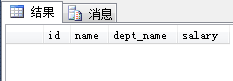• 插入元组

• insert into 关系名 values(每个属性对应的值)；
insert into instructor2 values(00001,'ai','math',2500.37);
insert into instructor2 values(00002,'la','math',3700.89);
insert into instructor2 values(00003,'bi','math',5400.45);
insert into instructor2 values(00004,'ma','english',1450.23);
insert into instructor2 values(00005,'ye','english',3421.34);
insert into instructor2 values(00006,'er','technology',10000.65);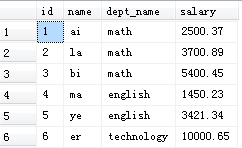• 删除所有元组

• delete from 关系名；
• 删除了元组，但关系和属性还存在。• 删除关系

• drop table 关系名；
• 删除了关系，进行select查询时会出现关系名无效的错误。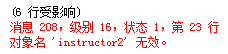• 属性操作

• alter table 关系名 add 新属性 数据类型，新属性 数据类型，$\cdots$$\cdots$

• 例：alter table instructor2 add firstname varchar(20),lastname varchar(20);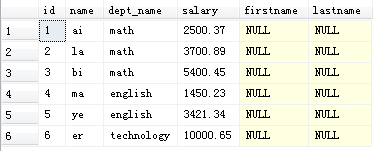• alter table 关系名 drop 属性，$\cdots$$\cdots$

• 例：alter table instructor2 drop firstname ,lastname ;
• 查询语句基础结构

• select对应投影$\mathrm{\Pi }$$\Pi$
• from对应笛卡尔积
• where对应选择$\sigma$$\sigma$
• 含义
• （1）.为from子句列出的关系产生笛卡尔积。
• （2）.在（1）的结果上应用where子句中指定的谓词（条件）。
• （3）.对于（2）中的元组，输出select子句中指定的属性。
select *(属性1，属性2，...)
from 关系名1，关系名2，...
where 条件1 and 条件2 and ...;

select id,name,salary
from instructor2
where salary>4000;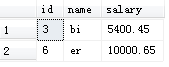• 去重操作（distinct）

• 对于一些属性来说，在某些元组中存在相同的值，有时我们需要对结果去重，得到正确答案。
select distinct 属性名
from 关系名
where 条件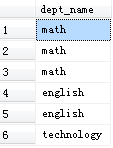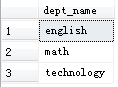• 更名操作（as）

• 优点
• 方便：长名字变短名字，便于书写语句。
• 同一关系笛卡尔积：区分属性。
• 对属性：
• 对关系：
select 属性 as 属性别名
from 关系名
where 条件
select 属性
from 关系名 as 关系别名
where 条件

select name as instructor_name,salary
from instructor2
where salary>3500;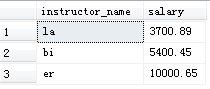select i1.id,i2.name
from instructor2 as i1,instructor2 as i2
where i1.id=i2.id and i2.salary>3500;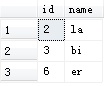• 字符串运算（like）

• SQL使用一对单引号来标示字符串，例‘math’。
• 百分号（%）：匹配任意子串
• 下划线（_）：匹配任意一个字符
select 属性
from 关系名
where 属性 like '字符形式';

select *
from instructor2
where salary like '%0_.%';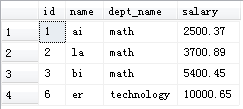• 显示次序（order by）

• 默认升序：asc
• 降序：desc
select 属性
from 关系名
where 条件
order by 属性 升序/降序；

select *
from instructor2
order by salary desc;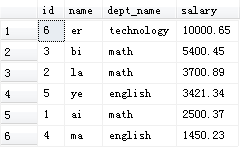• between$\cdots$$\cdots$and$\cdots$$\cdots$

• 属性值介于某个范围内
select *
from instructor2
where 属性名 between ... and ... ;

select *
from instructor2
where salary between 3000 and 6000;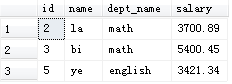• 分组聚集（group by）

• 聚集函数：平均值（avg）、最小值（min）、最大值（max）、总和（sum）、计数（count）。
• 出现在select语句中但没有被聚集的属性只能是出现在group by子句中的属性
• 错误查询
select dept_name,id,avg(salary)
from instructor2
group by dept_name;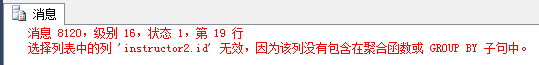• having子句（出现group by时使用）

• 有group by时，select 和 having子句中出现的属性有所限制
• 任何出现在having子句中，但没有被聚集的属性必须出现在group by子句中。
• 正确查询
select dept_name,avg(salary) as avg_salary
from instructor2
group by dept_name
having avg(salary)>3000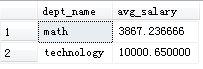• 集合成员资格

• in：测试元组是否是集合的成员
• not in：测试元组是否不是集合的成员
• in和 not in 操作符用于枚举集合
select *
from instructor2
where name not in('ai','ye','er');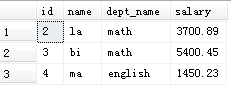select *
from instructor2
where name in (select name
from instructor2
where salary>3000
);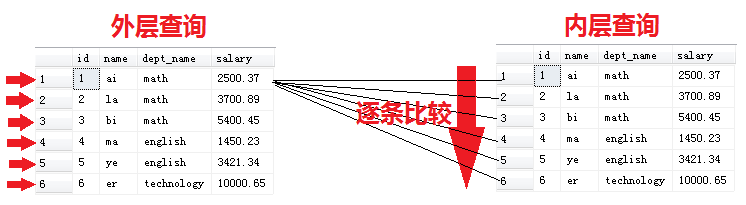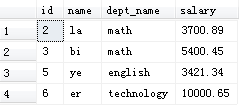• 集合的比较

• $>$$>$some：至少比某一个要大
• $>$$>$all：比所有的都大。
• $>$$>$some、$<$$<$some、$>=$$>=$some、$<=$$<=$some、$<>$$<>$some
• $>$$>$all、$<$$<$all、$>=$$>=$all、$<$$<$all、$<$$<$all
• $=$$=$some等价于in，$<>$$<>$some并不等价于not in
• $<>$$<>$all等价于not in，$=$$=$all不等价于in

select *
from instructor2
where name =some(select name
from instructor2
where salary>3000
);select *
from instructor2
where name <>all(select name
from instructor2
where salary>3000
);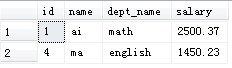select *
from instructor2
where name <>some(select name
from instructor2
where salary>3000
);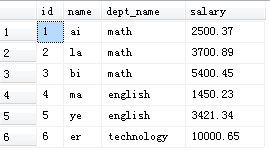select *
from instructor2
where name =all(select name
from instructor2
where salary>3000
);• 空关系测试（exists）

• 存在exists是相对于某一条元组，因而子查询中是select *

select course_id
from section as S
where semester='Fall' and year=2009 and exists(select *
from section as T
where semester='Spring' and year=2010
and S.course_id=T.course_id
);
• 删除某些元组

delete from instructor
where dept_name='math';
• 删除所有元组
delete from instructor;
• 删除的特殊情况

• 内层语句只有一条元组。

delete from instructor2
where salary < (
select avg(salary)
from instructor2
);

select *
from instructor2;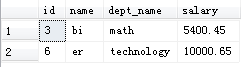• 插入一些元组

• insert into 关系名 values($\cdots$$\cdots$);一次只能插入一条元组。
• insert into 关系名 selec查询语句可一次性插入多条。

insert into instructor2
select id,name,dept_name,18000
from student
where dept_name='math';
• 更新元组
update 关系名
set 语句
where 条件；

update instructor2
set salary=salary*1.05;
where salary<7000;

update instructor2
set salary=case
when salary<=7000 then salary*1.05
else salary*1.03
end